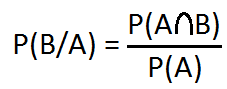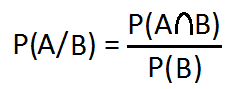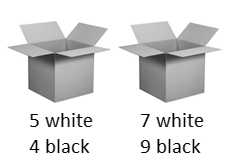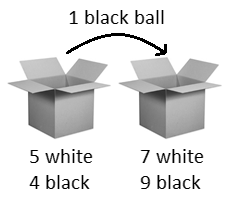# Conditional Probability

Aptitude

In this post we are going to discuss about conditional probability. To fully understand the topic you must have clear idea about permutation, combination and probability. If you want to revise or learn about these three topics before starting condition probability feel free to visit the links given below.

### Conditional Probability

Conditional probability deals with finding the probability of occurrence of an event provided some other event has already occurred.

Let A be an event associated with a random experiment and the number of favorable elementary events to the event A be N out of which the number of elementary events favorable to another event B is m. Then the conditional probability of B given A (i.e., under the condition that A has already occurred) is defined as the ratio of m/N and is denoted as P(B/A).

Note if N=0 i.e., if event A does not occur then the conditional probability P(B/A) cannot be defined.

## Formula for Conditional Probability

Let A and B be two events associated with a random experiment. Then the conditional probability of the occurrence of B given that A has already occurred isSimilarly, the conditional probability of the occurrence of A given that B has already occurred is## Red Ace

Q. What is the probability that the card draw from a deck of 52 cards is an ace given that the card is red?

A. This problem is an example of conditional probability. We are dealing with 52 cards and we know that there are 26 red cards and 26 black cards. According to the given problem it is given that a card is drawn and it is red in color. This is the condition. Now it is asked to find out the probability of an ace.

In this case the sample space does not consist of the outcomes of all 52 cards rather it deals with only 26 red cards and we know that there are only 2 red cards that are ace.

So the probability of drawing an ace given that the cards is red = P(ace/red) = 2/26 = 1/13

Alternatively you can solve this problem in the following way.

There are 26 red cards in a deck of 52 cards. So probability of selecting a red card from a deck of 52 cards is

P(red) = 26/52 = 1/2

There are 2 cards out of 52 cards that are both red and ace. So probability of selecting a card that is both red and ace is

P(red ∩ ace) = 2/52 = 1/26

So the probability of an ace given that the card drawn is red = P(ace/red)

P(ace/red) = P(red ∩ ace) / P(red) = (1/26) / (1/2) = 1/13

## Theorem

### Rule of Multiplication

Probability of the joint occurrence of A and B is
P(A∩B) = P(A).P(B/A)
P(A∩B) = P(B).P(A/B)

### Independent Events

Two events associated with a random experiment are said to be independent if the occurrence of one of the event does not affect the probability of occurrence of the other event.

If A and B be the two independent events then,
P(A/B) = P(A)

And
P(B/A) = P(B)

So we can say,
Two events A and B associated with a random experiment are said to be independent if and only if P(A∩B) = P(A).P(B)

### Dependent Events

If the occurrence and non-occurrence of one of the event affects the probability of the occurrence of the other event then the events are said to be dependent.

If two events A and B are dependent then,
P(A∩B) = P(A).P(B/A)
And
P(A∩B) = P(B).P(A/B)

Q. If two events A and B are independent and P(A) = 2/3 and P(B) = 3/5 then find P(A∪B)?

A. As the two given events A and B are independent so we get,
P(A∩B) = P(A).P(B)
= (2/3) . (3/5)
= 2/5
Therefore,
P(A∪B) = P(A) + P(B) – P(A∩B)
= 2/3 + 3/5 – 2/5 = 13/15

## Mars Mission

Q. 4 rovers are ready to be sent to land on Mars. ISRO has calculated the probability of success of each of the rovers to be 0.9. Find the probability of at least one them landing successfully?

A. Let A be the event “A rover will successful land on Mars”.
Then the probability of success is P(A) = 0.6
Then the probability that a rover will not land successfully is P(AC) = 1 – P(A) = 1 – 0.6 = 0.4
Probability that none of the 4 rovers will land = P(AC)4 = 0.44 = 0.0256
Therefore the probability that at least one of them will land successfully is
= 1 – Probability that none will succeed
= 1 – 0.0256
= 0.9744

## Even Balls

Q. A bag contains 20 balls numbered from 1 to 20. A ball is drawn at random and then another ball is drawn without replacement. Find the probability that both the balls are even numbered?

Note! Without replacement means you are not putting back the ball into the bag after drawing it out.

A. There are 20 balls numbered from 1 to 20. So there are 10 even numbered balls and 10 odd numbered balls.

Drawing the first even numbered ball:
Total number of elementary event favorable = 10
Size of the sample space = 20
Probability of selecting an even numbered ball = P(A) = 10/20

Drawing the second even numbered ball without replacement:
Total number of even balls left = 9
Size of the sample space after one ball is drawn = 19
Probability of drawing second even ball given the first ball was even= P(B/A) = 9/19

Hence the required probability that both the balls will be even = P(A).P(B/A) = 10/20 x 9/19 = 9/38

## Truth and Lie

Q. A speaks truth in 55% cases and B speaks truth in 75% cases. Find the percentage of cases they are likely to contradict each other in stating the fact?

A. From the given problem we get,
Probability of A speaking the truth = P(A) = 55/100
Probability of A lying = P(AC) = 1 – P(A) = 45/100
Probability of B speaking the truth = P(B) = 75/100
Probability of B lying = P(BC) = 1 – P(B) = 25/100

There exist two cases of contradictions
i. A speaks truth and B lies.
ii. A lies and B speaks the truth.

Case i
Probability = P(X1) = P(A) . P(BC)
= 55/100 x 25/100
= 11/80

Case ii
Probability = P(X2) = P(AC) . P(B)
= 45/100 x 75/100
= 27/80

So the total probability that A and B will contradict each other is
= 11/80 + 27/80
= 19/40

Therefore the required percentage = 47.5%

## Box

Q. A box contains 3 red and 5 blue balls. Two balls are drawn at random without replacement. Find the probability of getting 1 red and 1 blue ball?

A. Let R be the event “Red ball is drawn” and B be the event “Blue ball is drawn”.
According to the problem there are 3 red balls and 5 blue balls so there are total 8 balls in the box.
Probability of getting 1 red ball in the first draw = P(R) = 3/8
Since 1 ball is drawn out of the box so there are 7 balls left.
Probability of getting 1 blue ball in the second draw given that a red ball is already drawn = P(B/R) = 5/7

Therefore the required probability of drawing 1 red and 1 blue ball = P(R) . P(B/R) = 3/8 x 5/7 = 15/56

## Two Boxes

Q. One box contains 5 white and 4 black balls and the other box contains 7 white and 9 black balls. If a ball is drawn from the first box and put into the second box without replacement and then a ball is drawn from the second box. Find the probability that the ball drawing from the second box is white?A. There are two ways of transferring a ball from the first box to the second.

i. A white ball is transferred from the first box to the second.

ii. A black ball is transferred from the first box to the second.

Case i1 white ball can be selected out of 5 in 5C1 = 5 ways.
1 ball can be selected out of 9 balls in 9C1 = 9 ways.
Probability of transferring 1 white ball from the first to the second box = 5/9

After transferring 1 white ball, the second box contains 8 white balls and 9 black balls.
1 white ball can be selected out of 8 in 8C1 = 8 ways.
1 ball can be selected out of 17 balls in 17C1 = 17 ways.
Probability of drawing a white ball from the second box = 8/17

So probability of transferring 1 white ball from first box to the second and drawing 1 white ball from the second box = 5/9 x 8/17 = 40/153

Case ii1 black ball can be selected out of 4 in 4C1 = 4 ways.
1 ball can be selected out of 9 balls in 9C1 = 9 ways.
Probability of transferring 1 black ball from the first to the second box = 4/9

After transferring 1 black ball, the second box contains 7 white balls and 10 black balls.
1 white ball can be selected out of 7 in 7C1 = 7 ways.
1 ball can be selected out of 17 balls in 17C1 = 17 ways.
Probability of drawing a white ball from the second box = 7/17

So probability of transferring 1 black ball from first box to the second and drawing 1 white ball from the second box = 4/9 x 7/17 = 28/153

Therefore the required probability of drawing 1 white ball from the second box after a ball is transferred from the first box to the second

= 40/153 + 28/153
= 68/153
= 4/9

## Toss to win – Indefinite case

Q. A and B toss a coin alternatively till one of them gets a head and wins the game. Find their respective probability of winning, if A begins?

A. We know that the probability of getting a head by tossing = ½ and the probability of not getting a head = ½.
It is given that A will begin first.

Case 1: A winning the game

A will win the game if he gets head in the first toss. Or A fails in the first toss, B fails in the second toss and then A gets head in the third toss. Or A fails in the first, B fails in the second, A fails in the third, B fails in the fourth and then A gets head in the fifth toss. This may continue indefinitely.
Therefore the probability of A winning the game is
= ½ + (½ x ½ x ½) + (½ x ½ x ½ x ½ x ½) + …
= ½ + (½)3 + (½)5 + …
= (1/2)/(1 – (1/2)2) [using the formula of infinite GP series]
= 2/3

Case 2: B winning the game

B will win the game if A fails in the first toss and B wins the second toss. Or A fails in the first, B fails in the second, A fails in the third and B wins the fourth toss. Or A fails in the first toss, B fails in the second, A fails in the third, B fails in the fourth, A fails in the fifth and then B wins the sixth toss. This may continue indefinitely.
Therefore the probability of B winning the game is
= (½ x ½) + (½ x ½ x ½ x ½) + (½ x ½ x ½ x ½ x ½ x ½) + …
= (½)2 + (½)4 + (½)6 + …
= (½)2 / ( 1 - (½)2) [using the formula of infinite GP series]
= 1/3

There is an easy way to solve this problem and I think you have also guessed it out by now.
If you have calculated the probability of A winning the game then it becomes quite simple to find the probability of B winning the game by simply calculating probability of A not winning the game which is
= Probability of A not winning
= 1 – probability of A winning
= 1 – 2/3
= 1/3
So probability that B will win = 1/3